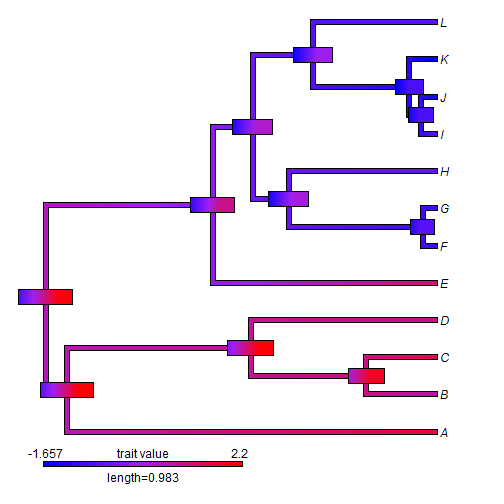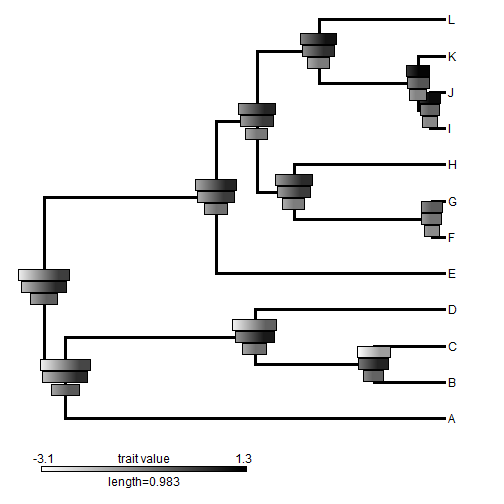## Monday, February 27, 2017

### More on adding error bars to a `"contMap"` plot

Earlier today I responded to a question about plotting color error bars at the internal nodes of a plotted `"contMap"` object. The original poster then also added that he would like to scale the length of the error bars by the degree of uncertainty in each estimated node state. This is fairly straightforward. Using the same tree & data we employed earlier, I would go about doing this as follows:

``````library(phytools)
aa<-fastAnc(tree,x,CI=TRUE)
xlim<-range(aa\$CI95)
obj<-contMap(tree,x,lims=xlim,plot=FALSE)
## for fun, let's change the color scheme:
obj<-setMap(obj,colors=c("blue","purple","red"))
plot(obj,xlim=c(-0.12,2.2))
d<-diff(obj\$lims)
v<-aa\$CI95[,2]-aa\$CI95[,1]
v<-v/max(v)
n<-length(obj\$cols)-1
lastPP<-get("last_plot.phylo",envir=.PlotPhyloEnv)
h<-max(nodeHeights(tree))
for(i in 1:tree\$Nnode){
ii<-round((aa\$CI95[i,1]-obj\$lims)/d*n)
jj<-round((aa\$CI95[i,2]-obj\$lims)/d*(n+1))
cols<-obj\$cols[ii:jj]
x=lastPP\$xx[i+Ntip(tree)]-0.05*h*v[i],
y=lastPP\$yy[i+Ntip(tree)],title="",subtitle="",lims=NULL,
lwd=14)
}
``````A second poster asked if it were possible to show multiple CIs in this way on a single tree. Of course - anything is possible, so why not give this a try too?

Here, I'll imagine I have the same tree as before, but three continuous characters in the columns of a data matrix `X` as follows:

``````X
``````
``````##           v1          v2         v3
## A -2.7149209 -1.60824200 -0.1530299
## B -2.6918371 -0.56868787 -0.4045761
## C -2.2214286  0.74691204 -0.7665039
## D -0.7471508 -0.07388221 -1.8163244
## E -0.2516396 -1.59142223 -0.7799235
## F -1.0164357 -0.77471225 -1.2468231
## G -0.2909680 -1.22294794 -1.2467781
## H -0.5319452 -0.10286165 -1.3781947
## I  1.0340376 -0.95934999 -0.9955973
## J  0.4739410 -0.49495658 -1.2955523
## K  1.1069054 -0.49403236 -1.1990746
## L -0.1095284  0.58439209 -1.3297114
``````

First, we need to repeat our analyses of above - but now across all three characters:

``````AA<-apply(X,2,fastAnc,tree=tree,CI=TRUE)
lims<-range(sapply(AA,function(x) range(x\$CI95)))
objs<-apply(X,2,contMap,tree=tree,lims=lims,plot=FALSE)
objs
``````
``````## \$v1
## Object of class "contMap" containing:
##
## (1) A phylogenetic tree with 12 tips and 11 internal nodes.
##
## (2) A mapped continuous trait on the range (-3.093201, 1.302283).
##
##
## \$v2
## Object of class "contMap" containing:
##
## (1) A phylogenetic tree with 12 tips and 11 internal nodes.
##
## (2) A mapped continuous trait on the range (-3.093201, 1.302283).
##
##
## \$v3
## Object of class "contMap" containing:
##
## (1) A phylogenetic tree with 12 tips and 11 internal nodes.
##
## (2) A mapped continuous trait on the range (-3.093201, 1.302283).
``````

Let's set our `"contMap"` objects to grey scale:

``````objs<-lapply(objs,setMap,colors=c("white","black"))
``````

For reasons that will become clear below, I'll also find the biggest CI on any node state across all three of the characters:

``````D<-sapply(AA,function(x) x\$CI95[,2]-x\$CI95[,1])
max.v<-max(D)
``````

Now, since we have three characters, maybe it makes more sense to plot the original tree & then just the CIs at nodes - along with our legend:

``````plotTree(tree,lwd=3,xlim=c(-0.12,2.16),ylim=c(-0.8,12))
lims=objs[]\$lims,prompt=FALSE,x=0,y=-0.4)
foo<-function(aa,obj,tree,y.shift,max.v){
d<-diff(obj\$lims)
n<-length(obj\$cols)-1
lastPP<-get("last_plot.phylo",envir=.PlotPhyloEnv)
h<-max(nodeHeights(tree))
v<-aa\$CI95[,2]-aa\$CI95[,1]
v<-v/max.v
for(i in 1:tree\$Nnode){
ii<-round((aa\$CI95[i,1]-obj\$lims)/d*n)
jj<-round((aa\$CI95[i,2]-obj\$lims)/d*(n+1))
cols<-obj\$cols[ii:jj]
x=lastPP\$xx[i+Ntip(tree)]-0.05*h*v[i],
y=lastPP\$yy[i+Ntip(tree)]+y.shift,
title="",subtitle="",lims=NULL,lwd=10)
}
}
for(i in 1:length(objs)) foo(AA[[i]],objs[[i]],tree,y.shift=(-i+2)/3,max.v=max.v)
``````That's it.